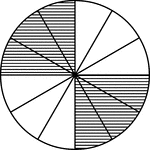### Fraction Pie Divided into Twelfths

A circle divided into twelfths with six twelfths shaded.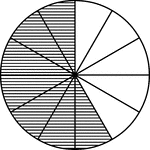### Fraction Pie Divided into Twelfths

A circle divided into twelfths with seven twelfths shaded.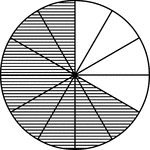### Fraction Pie Divided into Twelfths

A circle divided into twelfths with eight twelfths shaded.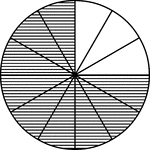### Fraction Pie Divided into Twelfths

A circle divided into twelfths with nine twelfths shaded.### Fraction Pie Divided into Twelfths

A circle divided into twelfths with ten twelfths shaded.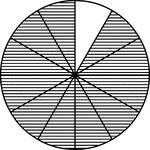### Fraction Pie Divided into Twelfths

A circle divided into twelfths with eleven twelfths shaded.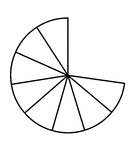### Eight Elevenths of a Fraction Pie

Eight elevenths of a circle.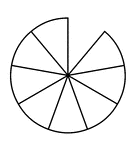### Eight Ninths of a Fraction Pie

Eight ninths of a circle.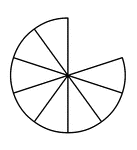### Eight Tenths of a Fraction Pie

Eight tenths of a circle.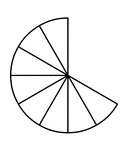### Eight Twelfths of a Fraction Pie

Eight twelfths of a circle.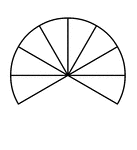### Eight Twelfths of a Fraction Pie

Eight twelfths of a circle.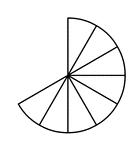### Eight Twelfths of a Fraction Pie

Eight twelfths of a circle.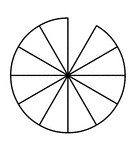### Eleven Twelfths of a Fraction Pie

Eleven twelfths of a circle.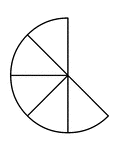### Five Eighths of a Fraction Pie

Five eighths of a circle.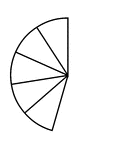### Five Elevenths of a Fraction Pie

Five elevenths of a circle.### Five Ninths of a Fraction Pie

Five ninths of a circle.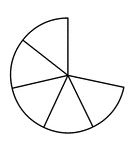### Five Sevenths of a Fraction Pie

Five sevenths of a circle.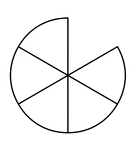### Five Sixths of a Fraction Pie

Five sixths of a circle.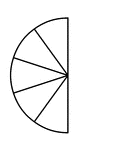### Five Tenths of a Fraction Pie

Five tenths of a circle.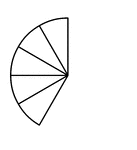### Five Twelfths of a Fraction Pie

Five twelfths of a circle.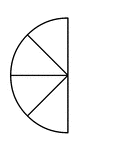### Four Eighths of a Fraction Pie

Four eighths of a circle.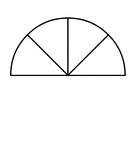### Four Eighths of a Fraction Pie

Four eighths of a circle.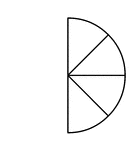### Four Eighths of a Fraction Pie

Four eighths of a circle.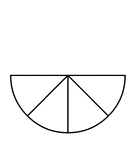### Four Eighths of a Fraction Pie

Four eighths of a circle.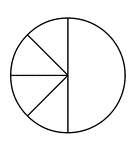### Four Eighths and One Half of a Pie Fraction

A circle subdivided into four eighths and one half.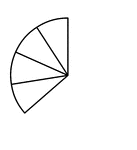### Four Elevenths of a Fraction Pie

Four elevenths of a circle.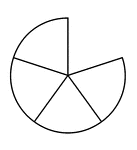### Four Fifths of a Fraction Pie

Four fifths of a circle.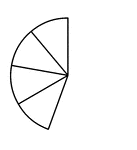### Four Ninths of a Fraction Pie

Four ninths of a circle.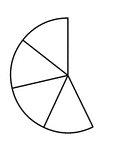### Four Sevenths of a Fraction Pie

Four sevenths of a circle.### Four Sixths of a Fraction Pie

Four sixths of a circle.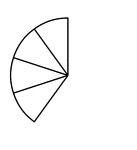### Four Tenths of a Fraction Pie

Four tenths of a circle.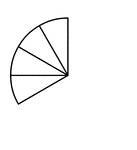### Four Twelfths of a Fraction Pie

Four twelfths of a circle.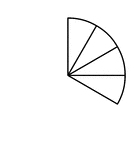### Four Twelfths of a Fraction Pie

Four twelfths of a circle.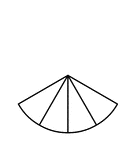### Four Twelfths of a Fraction Pie

Four twelfths of a circle.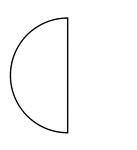### Half of a Fraction Pie

One half of a circle (vertical left).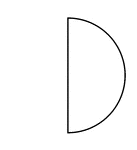### Half of a Fraction Pie

One half of a circle (vertical right).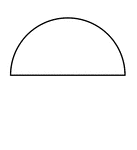### Half of a Fraction Pie

One half of a circle (horizontal top).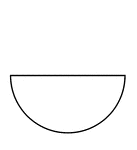### Half of a Fraction Pie

One half of a circle (horizontal bottom).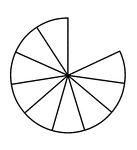### Nine Elevenths of a Fraction Pie

Nine elevenths of a circle.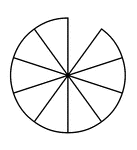### Nine Tenths of a Fraction Pie

Nine tenths of a circle.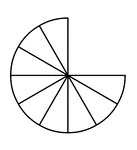### Nine Twelfths of a Fraction Pie

Nine twelfths of a circle.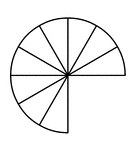### Nine Twelfths of a Fraction Pie

Nine twelfths of a circle.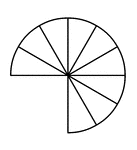### Nine Twelfths of a Fraction Pie

Nine twelfths of a circle.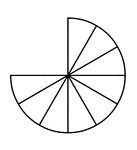### Nine Twelfths of a Fraction Pie

Nine twelfths of a circle.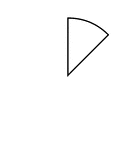### One Eighth of a Fraction Pie

One eighth of a circle.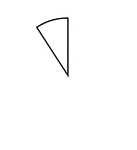### One Elevenths of a Fraction Pie

One eleventh of a circle.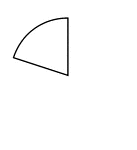### One Fifths of a Fraction Pie

One fifth of a circle.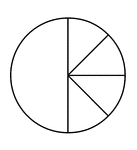### One Half and Four Eighths of a Pie Fraction

A circle subdivided into one half and four eighths.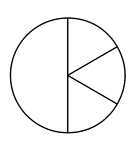### One Half and Three Sixths of a Pie Fraction

A circle subdivided into one half and three sixths.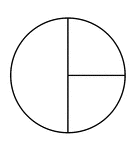### One Half and Two Quarters of a Pie Fraction

A circle subdivided into one half and two quarters.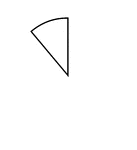### One Ninth of a Fraction Pie

One ninth of a circle.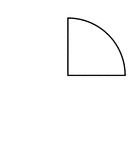### One Quarter of a Fraction Pie

One quarter of a circle.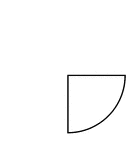### One Quarter of a Fraction Pie

One quarter of a circle.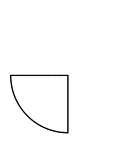### One Quarter of a Fraction Pie

One quarter of a circle.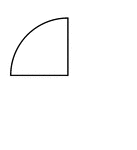### One Quarter of a Fraction Pie

One quarter of a circle.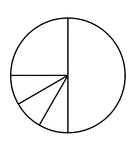### One Quarter, Three Twelfths, and One Half of a Pie Fraction

A circle subdivided into one quarter, three twelfths, and one half.### One Quarter, Two Eighths, and One Half of a Pie Fraction

A circle subdivided into one quarter, two eighths, and one half.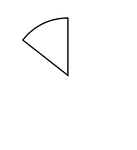### One Seventh of a Fraction Pie

One seventh of a circle.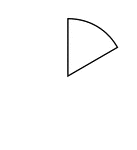### One Sixth of a Fraction Pie

One sixth of a circle.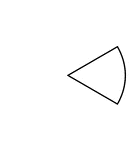### One Sixth of a Fraction Pie

One sixth of a circle.